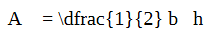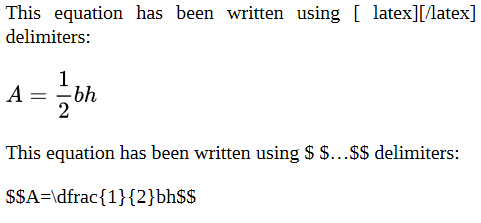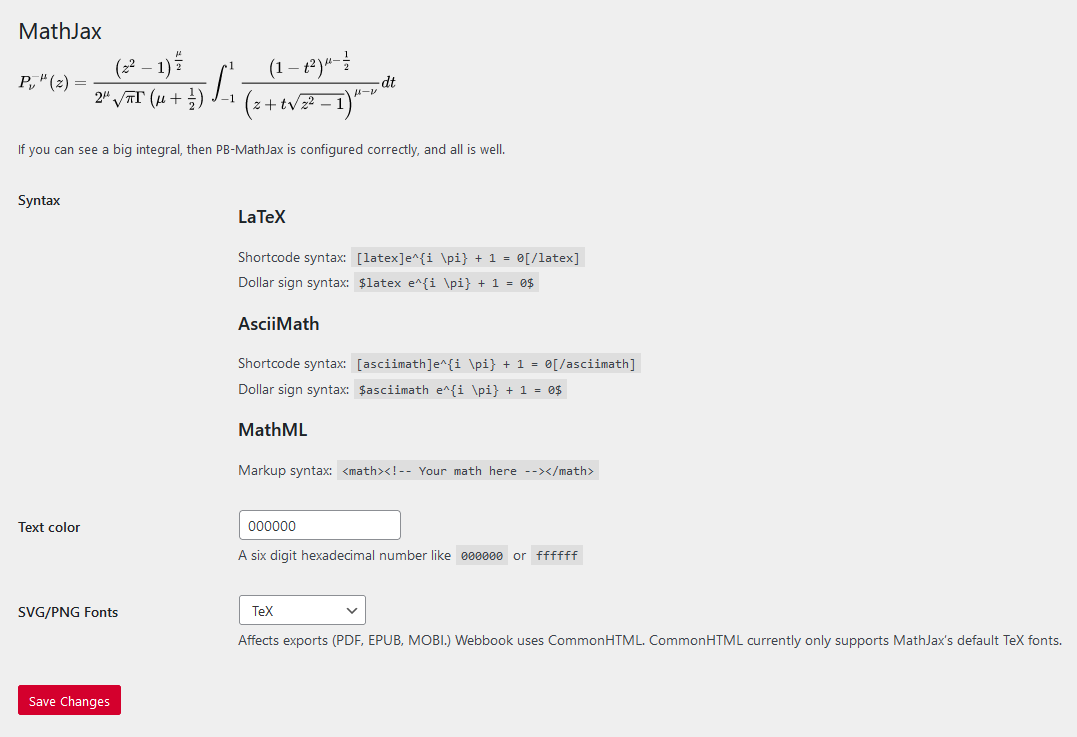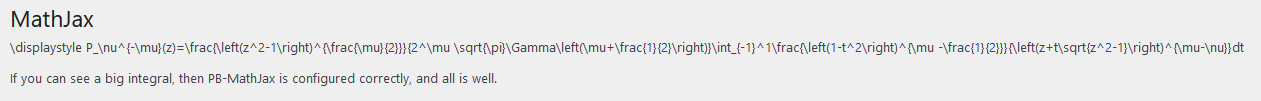# 55 Why won’t my LaTeX render properly in the PDF export of my book when it works fine in the web book?

Last update: Aug 8/22

PDFs in general can be finicky, and LaTeX is no exception to this. If your LaTeX is not displaying properly in a PDF, but renders just fine in the web book, one possible cause is excess spaces between commands: PDFs will often not display LaTeX formulas that are written with more than one consecutive space. (Note that this is only the case with LaTeX rendered with MathJax, not QuickLaTeX.)

For example, the equation `$A = \dfrac{1}{2} b h$` looks fine in the web book:

$A = \dfrac{1}{2} b h$

But here’s a screenshot of what it looks like in the PDF:As equations written in LaTeX are automatically spaced appropriately when rendered, it is not usually necessary to insert spaces into LaTeX commands. However, it is common to insert spaces to make equations more readable for humans working in the back end. If you choose to add single spaces between commands for your own readability as a LaTeX writer, be sure to search for excess spaces using the Find feature in your browser and delete them.

Another possible reason for LaTeX not rendering properly in a PDF export is the delimiters used to distinguish LaTeX from regular text.

There are two kinds of delimiters that can be used to mark the boundaries between LaTeX and and regular text: `$…$` and `$$…$$`. The former delimiters can be used for in-line equations (i.e., those that appear in the middle of a sentence), and the latter can be used for standalone equations that appear centred on their own line.

Unfortunately, equations written using `$$…$$` delimiters do not render in PDFs. Any LaTeX formulas you write using these delimiters may appear in the web book, but they will appear in the PDF exactly as they appear in editor view in Pressbooks.

To demonstrate, this equation has been written using `$…$` delimiters:

$A=\dfrac{1}{2}bh$

This equation has been written using `$$…$$` delimiters:

$$A=\dfrac{1}{2}bh$$

And here is a screenshot of a similar section of code in a PDF export:For this reason, it is best practice to only use `$…$` delimiters. If you would like your equation to appear centred and on its own line, simply write the equation in a new paragraph and manually centre it using the “Align centre” button or the shortcut `Shift + Alt + C`.

If there are no excess spaces in your commands and you’re using `$…$` delimiters, but your equations still are not rendering properly in the PDF, there may be a problem with MathJax, the engine that renders LaTeX in Pressbooks.

You can check to see if MathJax is working properly by going to Settings > MathJax on the Pressbooks dashboard. You should see a big integral at the top of the page, like this:The MathJax settings page says, “If you can see a big integral, then PB-MathJax [the MathJax plugin] is configured correctly, and all is well.”

However, if there’s a problem with MathJax, you may see this at the top of the page:If you see unrendered LaTeX instead of an integral, there is something wrong with the plugin for the BCcampus instance of Pressbooks. Please contact latexsupport@bccampus.ca as soon as possible to let us know about the problem.